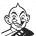Published:Updated:

# `Mental ability and basic numeracy..!' - From TNPSC to UPSC

`Mental ability and basic numeracy..!' - From TNPSC to UPSC

# `Mental ability and basic numeracy..!' - From TNPSC to UPSC

`Mental ability and basic numeracy..!' - From TNPSC to UPSC

Published:Updated:

Preliminary examination - Aptitude special - (Topic 2)

Next, in the aptitude paper, let us look at mental ability and basic numeracy. For answering these questions, it is not necessary to be a graduate or an expert in mathematics. Mostly the questions in this portion will be based on the lessons, theories and equations we have studied in school. Some of the major headings in these are given below.

Number row, number classification - integer, prime number, etc., with meanings and related questions, number divisibility, and associated basic topics.#### ஒட்டுமொத்த விகடனுக்கும் ஒரே ஷார்ட்கட்!

Sample question

#6897 is divisible by which of the following?

A. 11 only

C. 11 and 19

D. Neither 11 nor 19

Next, we will look at simplification, decimal fractions, average, square root, cube root, questions about quantity and age, percentage, profit, and loss, ratio, and proportion, partnership. Many questions come from these topics each year.

Sample question UPSC 2016

There is an order of 19000 quantity of a particular product from a customer. The firm produces 1000 the quantity of that product per out of which 5% are unfit for sale. In how many days will the order be completed?

(a) 18
(b) 19

(d) 22

Questions on time and work, time and distance, questions based on dimensions of pipes and buckets, questions on the speed of ships and trains, mixtures, simple interest and compound interest can be expected.

Sample question UPSC 2016

30g of sugar was mixed in 180 ml water in a vessel A, 40 g of sugar Was mixed in 280 ml of water in vessel B and 20 g of sugar was mixed in 100 ml of water in vessel C. The solution in vessel B is

(a) sweeter than that in C

(b) sweeter than that in A

(c) as sweet as that in C

(d) less sweet than that in C (answer)

Similarly, questions related to the area, volume, surface area, calendar, ratio, permutations, combination, probability, discount, heights, and distances need to be studied.

Sample question

X’s age is square of a number last year. Next year, his age is the cube of a number. After this, how many years does he have to
wait for his age to be the cube of a number again?

42